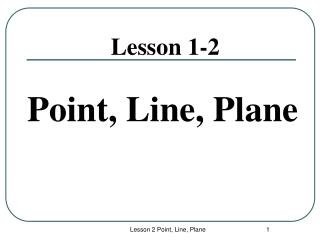DownloadDownload PresentationLesson 1-2

# Lesson 1-2

Download Presentation## Lesson 1-2

- - - - - - - - - - - - - - - - - - - - - - - - - - - E N D - - - - - - - - - - - - - - - - - - - - - - - - - - -
##### Presentation Transcript

1. Lesson 1-2 Point, Line, Plane Lesson 2 Point, Line, Plane

2. Points • Points do not have actual size. • How to Sketch: Using dots • How to label: Use capital letters Never name two points with the same letter (in the same sketch). A B C Lesson 2 Point, Line, Plane

3. Lines • Lines extend indefinitely and have no thickness or width. • How to sketch : using arrows at both ends. • How to name: 2 ways (1) small script letter – line n (2) any two points on the line - • Never name a line using three points - n A B C Lesson 2 Point, Line, Plane

4. Collinear Points • Collinear points are points that lie on the same line. (The line does not have to be visible.) • A point lies on the line if the coordinates of the point satisfy the equation of the line. Ex: To find if A (1, 0) is collinear with the points on the line y = -3x + 3. Substitute x = 1 and y = 0 in the equation. 0 = -3 (1) + 3 0 = -3 + 3 0 = 0 The point A satisfies the equation, therefore the point is collinear with the points on the line. A B C Collinear C A B Non collinear Lesson 2 Point, Line, Plane

5. Planes • A plane is a flat surface that extends indefinitely in all directions. • How to sketch: Use a parallelogram (four sided figure) • How to name: 2 ways (1) Capital script letter – Plane M (2) Any 3 non collinear points in the plane - Plane: ABC/ ACB / BAC / BCA / CAB / CBA A M B C Horizontal Plane Vertical Plane Other Lesson 2 Point, Line, Plane

6. Different planes in a figure: A B Plane ABCD Plane EFGH Plane BCGF Plane ADHE Plane ABFE Plane CDHG Etc. D C E F H G Lesson 2 Point, Line, Plane

7. Other planes in the same figure: Any three non collinear points determine a plane! Plane AFGD Plane ACGE Plane ACH Plane AGF Plane BDG Etc. Lesson 2 Point, Line, Plane

8. Coplanar Objects Coplanar objects (points, lines, etc.) are objects that lie on the same plane. The plane does not have to be visible. Are the following points coplanar? A, B, C ? Yes A, B, C, F ? No H, G, F, E ? Yes E, H, C, B ? Yes A, G, F ? Yes C, B, F, H ? No Lesson 2 Point, Line, Plane

9. Postulates or Axioms Definition: An assumption that needs no explanation. Accepted statement of fact Examples: • Postulate 1-1: Through any two points there is exactly one line. Lesson 2 Point, Line, Plane

10. Intersection of Figures The intersection of two figures is the set of points that are common in both figures. Postulate 1-2: The intersection of two lines is a point. m Line m and line n intersect at point P. P n Continued……. Lesson 2 Point, Line, Plane

11. Postulate 1-3: Intersection of Two Planes is a Line. R B P A Plane P and Plane R intersect at the line Lesson 2 Point, Line, Plane

12. 3 Possibilities of Intersection of a Line and a Plane (1) Line passes through plane – intersection is a point. (2) Line lies on the plane - intersection is a line. (3) Line is parallel to the plane - no common points. Lesson 2 Point, Line, Plane